Courses

# Doc: Kinetic Theory of Gases Class 11 Notes | EduRev

## Class 11 : Doc: Kinetic Theory of Gases Class 11 Notes | EduRev

The document Doc: Kinetic Theory of Gases Class 11 Notes | EduRev is a part of the Class 11 Course Chemistry Class 11.
All you need of Class 11 at this link: Class 11

The Kinetic Theory of Gases

In order to derive the theoretical aspect of the various gas laws based on simple experiment facts, Maxwell proposed the following postulates under the heading of kinetic theory of gases:

The postulates of kinetic theory of gas are

(i) Each gas is made up of a large number of small (tiny) particles known as molecules.

(ii) The volume of a molecule is so small that it may be neglected in comparison to total volume of gas.

(iii) The molecules are never in stationary state but they are believed to be in chaotic (random) motion. They travel in straight line in all possible directions with altogether different but constant velocities. The direction of motion is changed by the collision with container or with the other molecules.

(iv) The collision between molecules is perfectly elastic i.e., there is no change in the energies of the molecules after collision.

(v) The effect of gravity on molecular motion is negligible.

(vi) The kinetic energy of the gases depends on the temperature.

(vii) The pressure of the gas arises due to collision of molecules with the walls of the container.

The Kinetic Equation: Maxwell also derived an equation on the basis of above assumptions as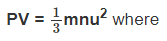P = Pressure of gas

V = Volume of gas

m = mass of one molecule of gas

n = no. of molecules of gas

u = root mean square velocity of molecules

For 1 mole n = N (Avogadro number)

m x N = Molecular mass M.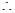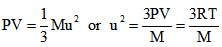or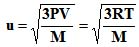Distribution of Molecular Velocities:

Maxwell and Boltzmann proposed that gas molecules are always in rapid random motion colliding with each other and with the walls of container. Due to such collisions, their velocities always changes. A fraction of molecules have a particular molecular velocity at a time. James Clark Maxwell calculated the distribution of velocity among fraction of total number of molecules, on the basis of probability.

The distribution of velocities of different gas molecules may be shown by the following curve.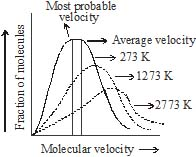From the curve it may be concluded that

(i) Only a small fraction of molecules have either very low or very high velocity.

(ii) Curve becomes flat when temperature is raised i.e. distribution around average velocity becomes wider. Average molecular velocity increases with rise in temperature.

(iii) Most of the molecules have velocity close to most probable velocity represented by the top of curve.

(iv) At higher temperature, greater number of molecules have high velocity, while few molecules have lower velocity.

Average Velocity :  As per kinetic theory of gases, each molecule is moving with altogether different velocity. Let `n' molecules be present in a given mass of gas, each one moving with velocity u1,u2, u3, …,un. The average velocity or Uav = average of all such velocity terms.

Average velocity =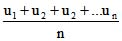Root Mean Square Velocity :  Maxwell proposed the term as the square root of means of square of all such velocities.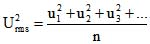Also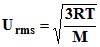Most probable velocity: It is the velocity possessed by maximum no. of molecules.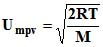Furthermore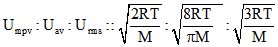=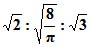= 1 : 1.128 : 1.224

Also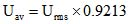Kinetic Energy of Gas: As per kinetic equation

For 1 mole m x n = Molecular Mass (M)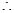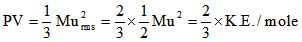or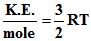Also KE per molecule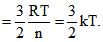Where k is the Boltzmann constant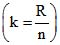Kinetic Energy of gas sample :

(i) Average kinetic energy of a single molecule =  T = KT

K = boltzman constant = 1.38 x 10-23 J/deg

(ii) total Kinetic Energy for one mole of gas =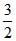RT

(iii) kinetic Energy for n mol of gas = n xRT

Illustration 13: Calculate rms speed of O2 at 273 K and 1 x 105 Pa pressure. The density of O2 under these conditions is 1.42 kg m-3

Solution: Data are given in SI units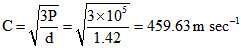Illustration 14: At what temperature will the r.m.s. velocity of oxygen be one and half times of its value at N.T.P.?

Solution: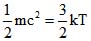Suppose the temperature required is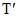then the velocity will be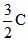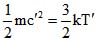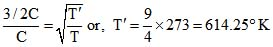Illustration 15: Calculate the average and total kinetic energy of 0.5 mole of an ideal gas at 0xC.

Solution:  Average kinetic energy =KT =x1.38 x10-23 x 273 = 5.65x 10-21

Total kinetic energy of n mole of gas = n xRT = 0.5 xx 8.314 x 273

= 1.702kJ

Offer running on EduRev: Apply code STAYHOME200 to get INR 200 off on our premium plan EduRev Infinity!

,

,

,

,

,

,

,

,

,

,

,

,

,

,

,

,

,

,

,

,

,

;